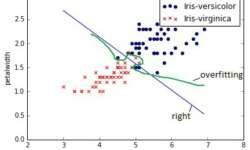## Regularized Logistic regressionPreviously we have tried logistic regression without regularization and with the simple training data set. But as we all know, things in real life aren’t as simple as we would want to. There are many types of data available that need to be classified. A number of features can grow up hundreds and thousands while a number of instances may be limited. Also in, many times, we might need to classify into more than two classes. The first problem that might arise due to many features is over-fitting. This is when learned hypothesis hΘ (x) fit training data too well (cost J(Θ) ≈ 0), but it fails when classifying new data samples. In other words, the model tries to distinct each training example correctly by drawing very complicated decision boundaries between training data points. As you can see in the image above, over-fitting would be green decision boundary. So how to deal with the over-fitting problem? There might be several approaches: We leave first two out of the question because selecting an optimal number of features is a different topic of optimization. Also, we are sticking with logistic regression model for now, so changing classifier is also out of the…